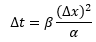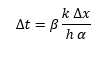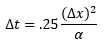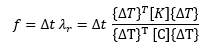you are here:/ News & Insights / Engineering Advantage Blog / Calculating Solution Settings for a Transient Thermal Analysis - Part 2

# Calculating Solution Settings for a Transient Thermal Analysis - Part 2May 24, 2016 By: Eric Stamper

Now that we are beyond the basics that were covered in the first part of this post, in this second part, we will address the calculation of the solution settings for this type of problem.

A transient thermal finite element analysis can be used to solve this type of problem and the simulation will produce a temperature field at a discrete number of points in time. The separation in time between these calculated sets of results is called the time step. The time step is important to both the accuracy and stability of the time marching solution procedure that is used to predict the temperature. Generally speaking, a smaller time step will produce a more accurate solution, but will increase overall runtime. Therefore, to approximate a reasonable time step size for a transient thermal analysis, both the Biot number and the thermal diffusivity can be used as shown in the following sets of equations:

If Bi < 1, the time step, Δt, can be calculated by:If Bi > 1, the time step, Δt, can be calculated by:Where: β=a scaling factor ranging from 0.1 to 0.5, Δx=element length at the location of the steepest thermal gradient of interest, α=thermal diffusivity, k=thermal conductivity, h= convective heat transfer coefficient.

The generalized trapezoidal rule for time integration is used by many FE codes, including ANSYS, to calculate the temperature field at each time point. This approach will calculate a result regardless of the time step size. However, the result will be incorrect if the time step is too large, and the errors will propagate in time.

In regions of severe thermal gradients during a transient (e.g., surfaces of quenched bodies), there is a relationship between the largest element size in the direction of the heat flow and the smallest time step size that will yield good results. Using more elements for the same time step size will normally give better results, but using a smaller time step for the same mesh will often give worse results. When using elements with midside nodes (quadratic elements), it is recommended to define the minimum time step size (or maximum element size) based on the following relationship:If the above relationship is violated when using elements with midside nodes, the program typically calculates unwanted oscillations and temperatures outside of the physically possible range.

A proper selection of the element size and time step size is therefore important for maintaining the accuracy of the solution that is calculated.

The oscillation limit, f, can be used to help gauge the accuracy of the transient response at each time point and is defined as:Where: Δt=the current time step, λ= Response Eigenvalue, {ΔT}= the change in temperature, [K]=conductivity matrix, [C]= specific heat matrix.

The oscillation limit is a measure of how well the response of the system is being captured. Thinking about the Response Eigenvalue in terms of an analogous structural quantity, it can be thought of as the natural frequency of the system (where the thermal quantities (K/C) are similar to stiffness (K) and mass (M) terms, e.g. f=√(K/M)).  In order to accurately capture the frequency of oscillation in a structural system, the sampling rate (in Hertz), fs=1/Δt, would need to be at least double the response frequency of interest (the minimum frequency is termed the Nyquist frequency, fN =0.5*fs)). As shown in Figure 1, below the system response of 0.1Hz is sampled at 1Hz, which provides an accurate representation of the response (equivalent to a β=0.1).

Figure 1: Discrete Representation of a Time Varying Response

## conduction4.pngThis same concept for the thermal problem, in terms of the oscillation limit, characterizes the relative rates of heat energy conduction and heat energy storage for a system. At each time step, the magnitude will indicate how well the response of the system is being captured (ANSYS directly outputs this value for each time step and similarly should be below 0.5 to insure accuracy as suggested by the upper limit of β=0.5).

I hope this overview helps to clarify a few aspects related to transient thermal problems and determining an appropriate solution time step size. I am curious to hear about any other comments you might have related to solving thermal analyses.